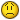# Loop to copy paste rows base on table arrays

#### Rusty867

Hi!

I'm fairly new to VBA and am having trouble with what I thought would be an easy loop. I'm trying to pull rows from a database dump, and copy paste them to a new sheet based on values of existing tables. Below is the code I have so far. I'm not getting any runtime errors, but after my routine runs nothing has been pasted over to new sheets I set up. Please help!Thanks in advance for any advise you could give..

Code:
``````Sub New_BG_Inventory()
'   Hide macro while working
Application.StatusBar = "Bringin' the Thunder..."
Application.ScreenUpdating = False

Sheets("Data").Select
Rows("1:6").Delete
'   Strip text label from CaseAge field value
Sheets("Data").Activate
Columns("C:C").Select
Selection.Insert Shift:=xlToRight, CopyOrigin:=xlFormatFromLeftOrAbove
Columns("B:B").Select
Selection.TextToColumns Destination:=Range("B1"), DataType:=xlDelimited, _
TextQualifier:=xlDoubleQuote, ConsecutiveDelimiter:=True, Tab:=False, _
Semicolon:=False, Comma:=False, Space:=True, Other:=False, FieldInfo _
:=Array(Array(1, 1), Array(2, 1)), TrailingMinusNumbers:=True
Columns("C:C").Delete
Columns("B:B").Select
Selection.NumberFormat = "0"

'   Declare and Set Navigation Variables
Dim valLR As Long
Dim valLC As Long
valLR = Cells(Rows.Count, 1).End(xlUp).Row
valLC = Cells(1, Columns.Count).End(xlToLeft).Column
Dim Nextrow As Long
Dim FinalCol As Long
FinalRow = Cells(Rows.Count, 1).End(xlUp).Row
Nextrow = Cells(Rows.Count, 1).End(xlUp).Row + 1

'   Declare and Set Report Variables
Dim PID As String
Dim PIDdata As String
Dim ROSTER As String
Dim ROSTERdata As String
Dim X As Variant
PID = Sheets("PIDs").Cells(FinalRow, 1)
ROSTER = Sheets("Roster").Cells(FinalRow, 2)

'   Loop for PID
Sheets("Data").Select

For X = 2 To FinalRow

PIDdata = Cells(X, 11).Value

If InStr(PID, PIDdata) > 0 And ROSTERdata <> "" Then

Sheets("PIDs").Select
Cells(X, 1).Resize(1, valLC + 10).Copy

Sheets("PID Rollup").Select
Nextrow = Cells(Rows.Count, 1).End(xlUp).Row + 1
Cells(Nextrow, 1).Select
ActiveSheet.Paste

Cells(X, 26).Select
ActiveCell.Formula = _
"=VLOOKUP(RC[-15],'PIDs'!R1C1:R1000C6,6,0)"
Cells(X, 27).Select
ActiveCell.FormulaR1C1 = "Primary"

Sheets("Data").Select
End If

Next X

'   Loop for Roster
Sheets("Data").Select
For X = 2 To FinalRow

ROSTERdata = Cells(X, 22).Value

If InStr(ROSTER, ROSTERdata) > 0 And ROSTERdata <> "" Then

Sheets("Roster").Select
Cells(X, 1).Resize(1, valLC + 10).Copy

Sheets("ROSTER Rollup").Select
Nextrow = Cells(Rows.Count, 1).End(xlUp).Row + 1
Cells(Nextrow, 1).Select
ActiveSheet.Paste

Cells(X, 26).Select
ActiveCell.Formula = _
"=VLOOKUP(RC[-4],'Roster'!R2C1:R200C2,2,0)"
Cells(X, 27).Select
ActiveCell.FormulaR1C1 = "Primary"

Sheets("Data").Select
End If

Next X

'   Hide Sheets
Sheets("PIDs").Select
ActiveWindow.SelectedSheets.Visible = False
Sheets("Roster").Select
ActiveWindow.SelectedSheets.Visible = False
Sheets("Data").Select
ActiveWindow.SelectedSheets.Visible = False

Application.StatusBar = ""
Application.ScreenUpdating = True

End Sub``````

Last edited: